# Mean State

Period Mean (original grids) [Watt m-2]
Model Period Mean (intersection) [Watt m-2]
Model Period Mean (complement) [Watt m-2]
Benchmark Period Mean (intersection) [Watt m-2]
Benchmark Period Mean (complement) [Watt m-2]
Bias [Watt m-2]
RMSE [Watt m-2]
Phase Shift [months]
Bias Score 
RMSE Score 
Seasonal Cycle Score 
Spatial Distribution Score 
Interannual Variability Score 
Overall Score 
Benchmark [-] 217.
CLM4 [-] 228. 227. 0.00 217. 232. 11.0 22.1 0.909 0.58 0.47 0.87 0.99 0.52 0.65
CLM4.5 [-] 228. 227. 0.00 217. 232. 11.0 22.1 0.909 0.58 0.47 0.87 0.99 0.52 0.65
CLM5 [-] 228. 227. 0.00 217. 232. 11.0 22.1 0.909 0.58 0.47 0.87 0.99 0.52 0.65
Period Mean (original grids) [Watt m-2]
Model Period Mean (intersection) [Watt m-2]
Model Period Mean (complement) [Watt m-2]
Benchmark Period Mean (intersection) [Watt m-2]
Benchmark Period Mean (complement) [Watt m-2]
Bias [Watt m-2]
RMSE [Watt m-2]
Phase Shift [months]
Bias Score 
RMSE Score 
Seasonal Cycle Score 
Spatial Distribution Score 
Interannual Variability Score 
Overall Score 
Benchmark [-] 241.
CLM4 [-] 245. 245. 0.00 241. 238. 4.28 22.8 0.581 0.65 0.52 0.95 0.93 0.66 0.71
CLM4.5 [-] 245. 245. 0.00 241. 238. 4.28 22.8 0.581 0.65 0.52 0.95 0.93 0.66 0.71
CLM5 [-] 245. 245. 0.00 241. 238. 4.28 22.8 0.581 0.65 0.52 0.95 0.93 0.66 0.71
Period Mean (original grids) [Watt m-2]
Model Period Mean (intersection) [Watt m-2]
Model Period Mean (complement) [Watt m-2]
Benchmark Period Mean (intersection) [Watt m-2]
Benchmark Period Mean (complement) [Watt m-2]
Bias [Watt m-2]
RMSE [Watt m-2]
Phase Shift [months]
Bias Score 
RMSE Score 
Seasonal Cycle Score 
Spatial Distribution Score 
Interannual Variability Score 
Overall Score 
Benchmark [-] 233.
CLM4 [-] 231. 231. 0.00 233. 238. -1.27 21.2 0.855 0.73 0.55 0.89 0.91 0.55 0.70
CLM4.5 [-] 231. 231. 0.00 233. 238. -1.27 21.2 0.855 0.73 0.55 0.89 0.91 0.55 0.70
CLM5 [-] 231. 231. 0.00 233. 238. -1.27 21.2 0.855 0.73 0.55 0.89 0.91 0.55 0.70
Period Mean (original grids) [Watt m-2]
Model Period Mean (intersection) [Watt m-2]
Model Period Mean (complement) [Watt m-2]
Benchmark Period Mean (intersection) [Watt m-2]
Benchmark Period Mean (complement) [Watt m-2]
Bias [Watt m-2]
RMSE [Watt m-2]
Phase Shift [months]
Bias Score 
RMSE Score 
Seasonal Cycle Score 
Spatial Distribution Score 
Interannual Variability Score 
Overall Score 
Benchmark [-] 111.
CLM4 [-] 112. 112. 0.00 111. 117. 0.518 16.3 0.0404 0.94 0.83 1.0 0.99 0.51 0.85
CLM4.5 [-] 112. 112. 0.00 111. 117. 0.518 16.3 0.0404 0.94 0.83 1.0 0.99 0.51 0.85
CLM5 [-] 112. 112. 0.00 111. 117. 0.518 16.3 0.0404 0.94 0.83 1.0 0.99 0.51 0.85
Period Mean (original grids) [Watt m-2]
Model Period Mean (intersection) [Watt m-2]
Model Period Mean (complement) [Watt m-2]
Benchmark Period Mean (intersection) [Watt m-2]
Benchmark Period Mean (complement) [Watt m-2]
Bias [Watt m-2]
RMSE [Watt m-2]
Phase Shift [months]
Bias Score 
RMSE Score 
Seasonal Cycle Score 
Spatial Distribution Score 
Interannual Variability Score 
Overall Score 
Benchmark [-] 213.
CLM4 [-] 216. 215. 0.00 209. 225. 9.33 24.3 1.51 0.56 0.43 0.78 0.91 0.49 0.60
CLM4.5 [-] 216. 215. 0.00 209. 225. 9.33 24.3 1.51 0.56 0.43 0.78 0.91 0.49 0.60
CLM5 [-] 216. 215. 0.00 209. 225. 9.33 24.3 1.51 0.56 0.43 0.78 0.91 0.49 0.60
Period Mean (original grids) [Watt m-2]
Model Period Mean (intersection) [Watt m-2]
Model Period Mean (complement) [Watt m-2]
Benchmark Period Mean (intersection) [Watt m-2]
Benchmark Period Mean (complement) [Watt m-2]
Bias [Watt m-2]
RMSE [Watt m-2]
Phase Shift [months]
Bias Score 
RMSE Score 
Seasonal Cycle Score 
Spatial Distribution Score 
Interannual Variability Score 
Overall Score 
Benchmark [-] 126.
CLM4 [-] 134. 134. 0.00 126. 145. 7.98 18.7 0.157 0.89 0.82 0.99 1.0 0.58 0.85
CLM4.5 [-] 134. 134. 0.00 126. 145. 7.98 18.7 0.157 0.89 0.82 0.99 1.0 0.58 0.85
CLM5 [-] 134. 134. 0.00 126. 145. 7.98 18.7 0.157 0.89 0.82 0.99 1.0 0.58 0.85
Period Mean (original grids) [Watt m-2]
Model Period Mean (intersection) [Watt m-2]
Model Period Mean (complement) [Watt m-2]
Benchmark Period Mean (intersection) [Watt m-2]
Benchmark Period Mean (complement) [Watt m-2]
Bias [Watt m-2]
RMSE [Watt m-2]
Phase Shift [months]
Bias Score 
RMSE Score 
Seasonal Cycle Score 
Spatial Distribution Score 
Interannual Variability Score 
Overall Score 
Benchmark [-] 170.
CLM4 [-] 188. 187. 0.00 170. 169. 16.8 27.8 0.502 0.72 0.74 0.96 0.89 0.62 0.78
CLM4.5 [-] 188. 187. 0.00 170. 169. 16.8 27.8 0.502 0.72 0.74 0.96 0.89 0.62 0.78
CLM5 [-] 188. 187. 0.00 170. 169. 16.8 27.8 0.502 0.72 0.74 0.96 0.89 0.62 0.78
Period Mean (original grids) [Watt m-2]
Model Period Mean (intersection) [Watt m-2]
Model Period Mean (complement) [Watt m-2]
Benchmark Period Mean (intersection) [Watt m-2]
Benchmark Period Mean (complement) [Watt m-2]
Bias [Watt m-2]
RMSE [Watt m-2]
Phase Shift [months]
Bias Score 
RMSE Score 
Seasonal Cycle Score 
Spatial Distribution Score 
Interannual Variability Score 
Overall Score 
Benchmark [-] 210.
CLM4 [-] 231. 231. 0.00 209. 224. 21.5 32.3 0.302 0.56 0.59 0.98 0.99 0.54 0.71
CLM4.5 [-] 231. 231. 0.00 209. 224. 21.5 32.3 0.302 0.56 0.59 0.98 0.99 0.54 0.71
CLM5 [-] 231. 231. 0.00 209. 224. 21.5 32.3 0.302 0.56 0.59 0.98 0.99 0.54 0.71
Period Mean (original grids) [Watt m-2]
Model Period Mean (intersection) [Watt m-2]
Model Period Mean (complement) [Watt m-2]
Benchmark Period Mean (intersection) [Watt m-2]
Benchmark Period Mean (complement) [Watt m-2]
Bias [Watt m-2]
RMSE [Watt m-2]
Phase Shift [months]
Bias Score 
RMSE Score 
Seasonal Cycle Score 
Spatial Distribution Score 
Interannual Variability Score 
Overall Score 
Benchmark [-] 105.
CLM4 [-] 103. 104. 0.00 105. 108. -1.87 18.0 0.284 0.92 0.80 0.98 0.90 0.52 0.82
CLM4.5 [-] 103. 104. 0.00 105. 108. -1.87 18.0 0.284 0.92 0.80 0.98 0.90 0.52 0.82
CLM5 [-] 103. 104. 0.00 105. 108. -1.87 18.0 0.284 0.92 0.80 0.98 0.90 0.52 0.82
Period Mean (original grids) [Watt m-2]
Model Period Mean (intersection) [Watt m-2]
Model Period Mean (complement) [Watt m-2]
Benchmark Period Mean (intersection) [Watt m-2]
Benchmark Period Mean (complement) [Watt m-2]
Bias [Watt m-2]
RMSE [Watt m-2]
Phase Shift [months]
Bias Score 
RMSE Score 
Seasonal Cycle Score 
Spatial Distribution Score 
Interannual Variability Score 
Overall Score 
Benchmark [-] 228.
CLM4 [-] 235. 235. 0.00 229. 216. 2.90 18.7 0.429 0.88 0.74 0.97 0.97 0.65 0.82
CLM4.5 [-] 235. 235. 0.00 229. 216. 2.90 18.7 0.429 0.88 0.74 0.97 0.97 0.65 0.82
CLM5 [-] 235. 235. 0.00 229. 216. 2.90 18.7 0.429 0.88 0.74 0.97 0.97 0.65 0.82
Period Mean (original grids) [Watt m-2]
Model Period Mean (intersection) [Watt m-2]
Model Period Mean (complement) [Watt m-2]
Benchmark Period Mean (intersection) [Watt m-2]
Benchmark Period Mean (complement) [Watt m-2]
Bias [Watt m-2]
RMSE [Watt m-2]
Phase Shift [months]
Bias Score 
RMSE Score 
Seasonal Cycle Score 
Spatial Distribution Score 
Interannual Variability Score 
Overall Score 
Benchmark [-] 140.
CLM4 [-] 158. 158. 0.00 138. 161. 20.2 28.5 0.194 0.75 0.80 0.99 1.0 0.64 0.83
CLM4.5 [-] 158. 158. 0.00 138. 161. 20.2 28.5 0.194 0.75 0.80 0.99 1.0 0.64 0.83
CLM5 [-] 158. 158. 0.00 138. 161. 20.2 28.5 0.194 0.75 0.80 0.99 1.0 0.64 0.83
Period Mean (original grids) [Watt m-2]
Model Period Mean (intersection) [Watt m-2]
Model Period Mean (complement) [Watt m-2]
Benchmark Period Mean (intersection) [Watt m-2]
Benchmark Period Mean (complement) [Watt m-2]
Bias [Watt m-2]
RMSE [Watt m-2]
Phase Shift [months]
Bias Score 
RMSE Score 
Seasonal Cycle Score 
Spatial Distribution Score 
Interannual Variability Score 
Overall Score 
Benchmark [-] 191.
CLM4 [-] 190. 190. 0.00 186. 193. 4.01 23.2 0.455 0.76 0.69 0.95 0.98 0.54 0.77
CLM4.5 [-] 190. 190. 0.00 186. 193. 4.01 23.2 0.455 0.76 0.69 0.95 0.98 0.54 0.77
CLM5 [-] 191. 191. 0.00 186. 193. 4.01 23.2 0.455 0.76 0.69 0.95 0.98 0.54 0.77
Period Mean (original grids) [Watt m-2]
Model Period Mean (intersection) [Watt m-2]
Model Period Mean (complement) [Watt m-2]
Benchmark Period Mean (intersection) [Watt m-2]
Benchmark Period Mean (complement) [Watt m-2]
Bias [Watt m-2]
RMSE [Watt m-2]
Phase Shift [months]
Bias Score 
RMSE Score 
Seasonal Cycle Score 
Spatial Distribution Score 
Interannual Variability Score 
Overall Score 
Benchmark [-] 240.
CLM4 [-] 249. 249. 0.00 240. 241. 8.59 22.4 0.367 0.81 0.74 0.97 0.99 0.61 0.81
CLM4.5 [-] 249. 249. 0.00 240. 241. 8.59 22.4 0.367 0.81 0.74 0.97 0.99 0.61 0.81
CLM5 [-] 249. 249. 0.00 240. 241. 8.59 22.4 0.367 0.81 0.74 0.97 0.99 0.61 0.81
Period Mean (original grids) [Watt m-2]
Model Period Mean (intersection) [Watt m-2]
Model Period Mean (complement) [Watt m-2]
Benchmark Period Mean (intersection) [Watt m-2]
Benchmark Period Mean (complement) [Watt m-2]
Bias [Watt m-2]
RMSE [Watt m-2]
Phase Shift [months]
Bias Score 
RMSE Score 
Seasonal Cycle Score 
Spatial Distribution Score 
Interannual Variability Score 
Overall Score 
Benchmark [-] 113.
CLM4 [-] 117. 117. 0.00 113. 116. 3.88 18.4 0.110 0.89 0.82 0.99 0.79 0.48 0.80
CLM4.5 [-] 117. 117. 0.00 113. 116. 3.88 18.4 0.110 0.89 0.82 0.99 0.79 0.48 0.80
CLM5 [-] 117. 117. 0.00 113. 116. 3.88 18.4 0.110 0.89 0.82 0.99 0.79 0.48 0.80
Period Mean (original grids) [Watt m-2]
Model Period Mean (intersection) [Watt m-2]
Model Period Mean (complement) [Watt m-2]
Benchmark Period Mean (intersection) [Watt m-2]
Benchmark Period Mean (complement) [Watt m-2]
Bias [Watt m-2]
RMSE [Watt m-2]
Phase Shift [months]
Bias Score 
RMSE Score 
Seasonal Cycle Score 
Spatial Distribution Score 
Interannual Variability Score 
Overall Score 
Benchmark [-] 229.
CLM4 [-] 223. 223. 0.00 227. 241. -2.57 26.4 0.757 0.67 0.63 0.92 0.70 0.57 0.69
CLM4.5 [-] 223. 223. 0.00 227. 241. -2.57 26.4 0.757 0.67 0.63 0.92 0.70 0.57 0.69
CLM5 [-] 223. 223. 0.00 227. 241. -2.57 26.4 0.757 0.67 0.63 0.92 0.70 0.57 0.69
Period Mean (original grids) [Watt m-2]
Model Period Mean (intersection) [Watt m-2]
Model Period Mean (complement) [Watt m-2]
Benchmark Period Mean (intersection) [Watt m-2]
Benchmark Period Mean (complement) [Watt m-2]
Bias [Watt m-2]
RMSE [Watt m-2]
Phase Shift [months]
Bias Score 
RMSE Score 
Seasonal Cycle Score 
Spatial Distribution Score 
Interannual Variability Score 
Overall Score 
Benchmark [-] 173.
CLM4 [-] 186. 186. 0.00 172. 207. 15.1 22.2 0.367 0.77 0.78 0.98 0.98 0.50 0.80
CLM4.5 [-] 186. 186. 0.00 172. 207. 15.1 22.2 0.367 0.77 0.78 0.98 0.98 0.50 0.80
CLM5 [-] 186. 186. 0.00 172. 207. 15.1 22.2 0.367 0.77 0.78 0.98 0.98 0.50 0.80
Period Mean (original grids) [Watt m-2]
Model Period Mean (intersection) [Watt m-2]
Model Period Mean (complement) [Watt m-2]
Benchmark Period Mean (intersection) [Watt m-2]
Benchmark Period Mean (complement) [Watt m-2]
Bias [Watt m-2]
RMSE [Watt m-2]
Phase Shift [months]
Bias Score 
RMSE Score 
Seasonal Cycle Score 
Spatial Distribution Score 
Interannual Variability Score 
Overall Score 
Benchmark [-] 135.
CLM4 [-] 149. 149. 0.00 135. 133. 13.8 20.4 0.186 0.83 0.82 0.99 0.96 0.54 0.83
CLM4.5 [-] 149. 149. 0.00 135. 133. 13.8 20.4 0.186 0.83 0.82 0.99 0.96 0.54 0.83
CLM5 [-] 149. 149. 0.00 135. 133. 13.8 20.4 0.186 0.83 0.82 0.99 0.96 0.54 0.83
Period Mean (original grids) [Watt m-2]
Model Period Mean (intersection) [Watt m-2]
Model Period Mean (complement) [Watt m-2]
Benchmark Period Mean (intersection) [Watt m-2]
Benchmark Period Mean (complement) [Watt m-2]
Bias [Watt m-2]
RMSE [Watt m-2]
Phase Shift [months]
Bias Score 
RMSE Score 
Seasonal Cycle Score 
Spatial Distribution Score 
Interannual Variability Score 
Overall Score 
Benchmark [-] 193.
CLM4 [-] 191. 191. 0.00 193. 181. -1.86 16.9 0.233 0.92 0.81 0.98 0.98 0.54 0.84
CLM4.5 [-] 191. 191. 0.00 193. 181. -1.86 16.9 0.233 0.92 0.81 0.98 0.98 0.54 0.84
CLM5 [-] 191. 191. 0.00 193. 181. -1.86 16.9 0.233 0.92 0.81 0.98 0.98 0.54 0.84
Period Mean (original grids) [Watt m-2]
Model Period Mean (intersection) [Watt m-2]
Model Period Mean (complement) [Watt m-2]
Benchmark Period Mean (intersection) [Watt m-2]
Benchmark Period Mean (complement) [Watt m-2]
Bias [Watt m-2]
RMSE [Watt m-2]
Phase Shift [months]
Bias Score 
RMSE Score 
Seasonal Cycle Score 
Spatial Distribution Score 
Interannual Variability Score 
Overall Score 
Benchmark [-] 202.
CLM4 [-] 211. 211. 0.00 201. 241. 10.1 21.6 1.24 0.61 0.46 0.83 0.91 0.51 0.63
CLM4.5 [-] 211. 211. 0.00 201. 241. 10.1 21.6 1.24 0.61 0.46 0.83 0.91 0.51 0.63
CLM5 [-] 211. 211. 0.00 201. 241. 10.1 21.6 1.24 0.61 0.46 0.83 0.91 0.51 0.63
Period Mean (original grids) [Watt m-2]
Model Period Mean (intersection) [Watt m-2]
Model Period Mean (complement) [Watt m-2]
Benchmark Period Mean (intersection) [Watt m-2]
Benchmark Period Mean (complement) [Watt m-2]
Bias [Watt m-2]
RMSE [Watt m-2]
Phase Shift [months]
Bias Score 
RMSE Score 
Seasonal Cycle Score 
Spatial Distribution Score 
Interannual Variability Score 
Overall Score 
Benchmark [-] 211.
CLM4 [-] 217. 217. 0.00 211. 216. 5.51 23.6 0.836 0.77 0.58 0.90 0.84 0.49 0.69
CLM4.5 [-] 217. 217. 0.00 211. 216. 5.51 23.6 0.836 0.77 0.58 0.90 0.84 0.49 0.69
CLM5 [-] 217. 217. 0.00 211. 216. 5.51 23.6 0.836 0.77 0.58 0.90 0.84 0.49 0.69
Period Mean (original grids) [Watt m-2]
Model Period Mean (intersection) [Watt m-2]
Model Period Mean (complement) [Watt m-2]
Benchmark Period Mean (intersection) [Watt m-2]
Benchmark Period Mean (complement) [Watt m-2]
Bias [Watt m-2]
RMSE [Watt m-2]
Phase Shift [months]
Bias Score 
RMSE Score 
Seasonal Cycle Score 
Spatial Distribution Score 
Interannual Variability Score 
Overall Score 
Benchmark [-] 204.
CLM4 [-] 210. 209. 0.00 205. 190. 1.38 32.0 0.437 0.70 0.69 0.96 0.76 0.52 0.72
CLM4.5 [-] 210. 209. 0.00 205. 190. 1.38 32.0 0.437 0.70 0.69 0.96 0.76 0.52 0.72
CLM5 [-] 210. 209. 0.00 205. 190. 1.38 32.0 0.437 0.70 0.69 0.96 0.76 0.52 0.72

# Temporally integrated period mean

BENCHMARK MEAN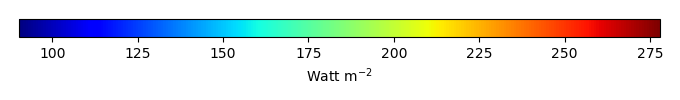MODEL MEANBIAS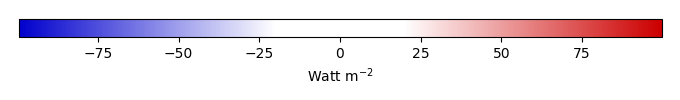BIAS SCORERMSE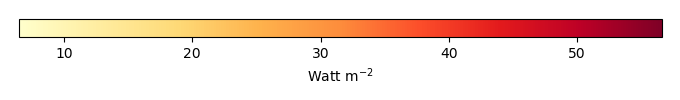RMSE SCOREBENCHMARK INTERANNUAL VARIABILITY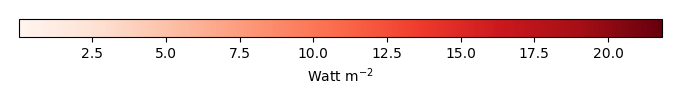MODEL INTERANNUAL VARIABILITYINTERANNUAL VARIABILITY SCOREBENCHMARK MAX MONTHMODEL MAX MONTHDIFFERENCE IN MAX MONTH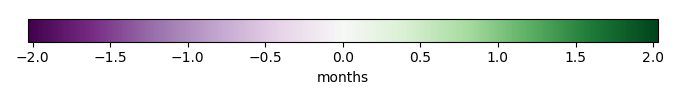SEASONAL CYCLE SCORESPATIAL TAYLOR DIAGRAMMODEL COLORS# Spatially integrated regional mean

MODEL COLORSREGIONAL MEANANNUAL CYCLEMONTHLY ANOMALYANNUAL CYCLE# All Models

BenchmarkCLM4CLM4.5CLM5# Data Information

creation_date: Mon Jun 30 23:16:59 PDT 2014

source_file: This product is generated from monthly 1 degree GEWEX SRB Radiation observations